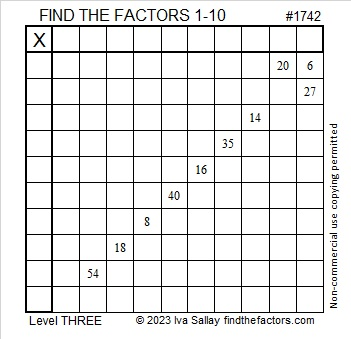# 1742 Just How Sharp Do You Think Cupid’s Arrow Is?

Contents

### Today’s Puzzle:

This puzzle looks a little like an arrow that Cupid might be aiming your way. I’m not sure how sharp it is, but here’s hoping that it’s not the least bit painful.

Since this is a level-3 puzzle, start with the clues at the top of the puzzle and work your way down cell by cell until you have found all the factors. Each number from 1 to 10 can only appear one time in the first column and one time in the top row. There is only one solution.### Factors of 1742:

• 1742 is a composite number.
• Prime factorization: 1742 = 2 × 13 × 67.
• 1742 has no exponents greater than 1 in its prime factorization, so √1742 cannot be simplified.
• The exponents in the prime factorization are 1, 1, and 1. Adding one to each exponent and multiplying we get (1 + 1)(1 + 1)(1 + 1) = 2 × 2 × 2 = 8. Therefore 1742 has exactly 8 factors.
• The factors of 1742 are outlined with their factor pair partners in the graphic below.### More About the Number 1742:

1742 is the hypotenuse of a Pythagorean triple:
670-1608-1742 which is (5-12-13) times 134.

1742 looks interesting in some other bases:
It’s 23432 in base 5 because 2(5⁴) + 3(5³) + 4(5²) + 3(5) + 2(1) = 1742,
2345 in base 9 because 2(9³) + 3(9²) + 4(9) + 5(1) = 1742, and
repdigit 222 in base 29 because 2(29² + 29 + 1) = 1742.

This site uses Akismet to reduce spam. Learn how your comment data is processed.